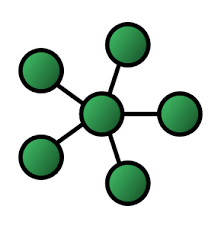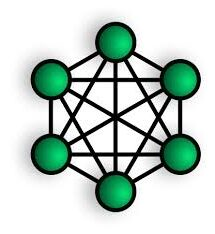# Network Topology – Meaning and Types of Network Topology (Continuation) Primary 6 (Basic 6) – Computer Studies

### COMPUTER STUDIES

BASIC SCIENCE AND TECHNOLOGY

FIRST TERM

WEEK 5

PRIMARY 6

THEME – BASIC COMPUTER OPERATION

PREVIOUS LESSON – Network Topology – Meaning and Types Network Topology Primary 6 (Basic 6) – Computer Studies

### LEARNING AREA

1. Introductory Activities

2. Meaning of Network Topology

3. Types of Network Topology

4. Stretch Different Types of Network Topology

### PERFORMANCE OBJECTIVES

By the end of the lesson, most of the pupils should have attained the following objectives –

1. describe network topology;

2. state types of network topology;

3. sketch and describe bus, ring and star topology.

### ENTRY BEHAVIOR

The pupils can state the requirements for computer network.

### INSTRUCTIONAL MATERIALS

The teacher will teach the lesson with the aid of charts of network topology.

### METHOD OF TEACHING

Choose a suitable and appropriate methods for the lessons.

Note – Irrespective of choosing methods of teaching, always introduce an activities that will arouse pupil’s interest or lead them to the lessons.

### REFERENCE MATERIALS

Scheme of Work

9 – Years Basic Education Curriculum

Course Book – Tanus Computer Studies book 6

All Relevant Material

Online Information

### LESSON 1 – INTRODUCTION

Network topology is the arrangement and description of a computer network.

TYPES OF NETWORK TOPOLOGY

1. Bus topology

2. Ring topology

3. Star topology

4. Mash topology

5. Tree topology

6. Point to point topology

STAR TOPOLOGY

Star topology is a network where all computer are connected at a central point.MESH TOPOLOGY

Mesh topology is a network where all the computer are interconnected with one another.TREE TOPOLOGY

Tree topology is a network where all the computers are connected like a branches of a tree.POINT TO POINT TOPOLOGY

Point to point topology is a network where two or more computer are connected to one another direct in a line.### PRESENTATION

To deliver the lesson, the teacher adopts the following steps:

1. Revises the previous lesson based on the pupil’s related knowledge or experience.

Pupil’s Activities – Participate actively in the lesson review.

2. Displays charts showing types of network topology.

3. Lets pupils different each of the network based on the arrangement.

Pupil’s Activities – Identify the setup of each network topology.

4. Uses the chart to introduce and explain each type of topology.

Pupil’s Activities – Listen to the teacher’s explanation.

5. Gets two or more functional computers to illustration each topology. If available

Pupil’s Activities – Support the teacher in setup each network topology.

6. Sketch and ask pupils to sketch each network topology.

Pupil’s Activities – Sketch all the network topology.

7. Summarizes the lesson on the board.

Pupil’s Activities – Copy as instructed.

### CONCLUSION

• To conclude the lesson for the week, the teacher revises the entire lesson and links it to the following week’s lesson.

### LESSON EVALUATION

1. Explain the meaning of network topology.

2. List and explain any 3 network topology with appropriate drawing.

### WEEKLY ASSESSMENT

1. ________ is the arrangement and description of a computer network.

A. Computer network

B. Computer system

C. Computer network topology

D. Computer hardware

2. There are ________ types of network topology.

A. 3

B. 4

C. 5

D. 6

3. ________ types of network topology looks like a circle.

A. Bus topology

B. Ring topology

C. Star topology

D. Circle topology

4. ________ types of network topology looks like a sitting bus.

A. Bus topology

B. Ring topology

C. Star topology

D. Mesh topology

5. ________ types of network topology looks like a star.

A. Bus topology

B. Ring topology

C. Star topology

D. Circle topology

6. Network topology is the ________ and ________ of a computer network.

7. ________ is a type of network where all the computers are connected to a single cable.

A. Point to point topology

B. Ring topology

C. Bus topology

D. Mesh topology

8. ________ is a type of network where  all computer are connected to create a circular data path.

A. Bus topology

B. Ring topology

C. Star topology

D. Mesh topology

9. ________ is a network where all computer are connected at a central point.

A. Bus topology

B. Star topology

C. Tree topology

D. Ring topology

10. ________ is a network where all the computer are interconnected with one another.

A. Mesh topology

B. Ring topology

C. Star topology

D. Bus topology

11. ________ is a network where all the computers are connected like a branches of a tree.

A. Point to point topology

B. Ring topology

C. Tree topology

D. Mesh topology

12. ________ is a network where two or more computer are connected to one another direct in a line.

A. Point to point topology

B. Ring topology

C. Bus topology

D. Mesh topology

13. Bus topology is a network where two or more computer are connected to one another direct in a line.

A. True

B. False

14. Star topology is a network where all the computers are connected like a branches of a tree.

A. False

B. True

15. Mesh topology is a network where all the computer are interconnected with one another.

A. False

B. True

16. Bus topology is a type of network where all the computers are connected to a single cable.

A. False

B. True

17. Sketch a mesh topology.

18. Sketch a star topology.

19. Sketch a bus topology.

20. Sketch a tree topology.